# Chapter 2 Elasticity and Viscoelasticity Mechanical Testing Machine

• Slides: 39Chapter 2 Elasticity and Viscoelasticity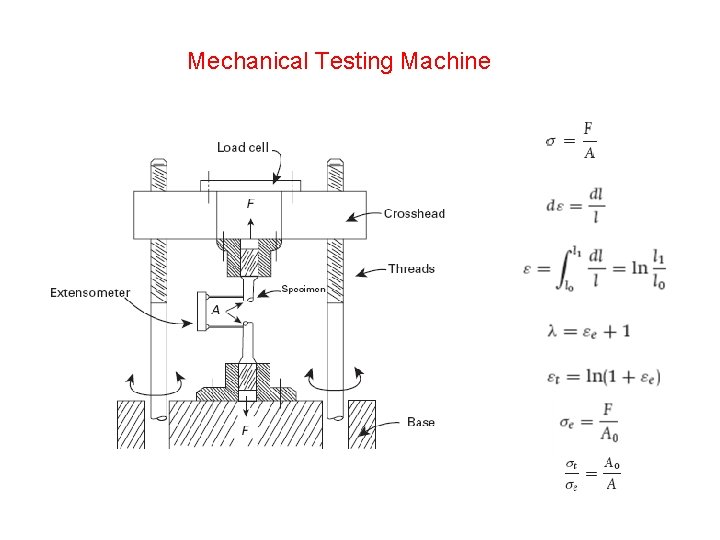Mechanical Testing MachineElastic Behavior Stress–strain curves in an elastic regime. (a) Linear elastic curve , typical for metals, ceramics, and some polymers. (b) Nonlinear elastic curve, typical for rubber.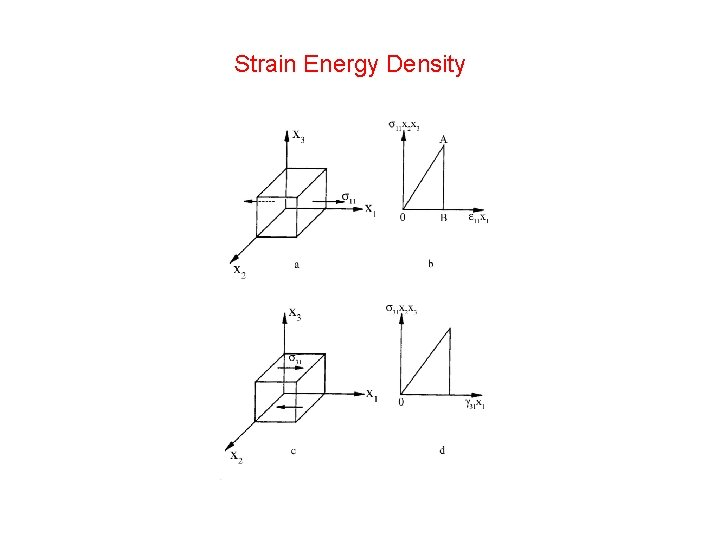Strain Energy DensityShear Stress and Shear Strain (a) Specimen subjected to shear force. (b) Strain undergone by small cube in shear region. (c) Specimen (cylinder) subjected to torsion by a torque T.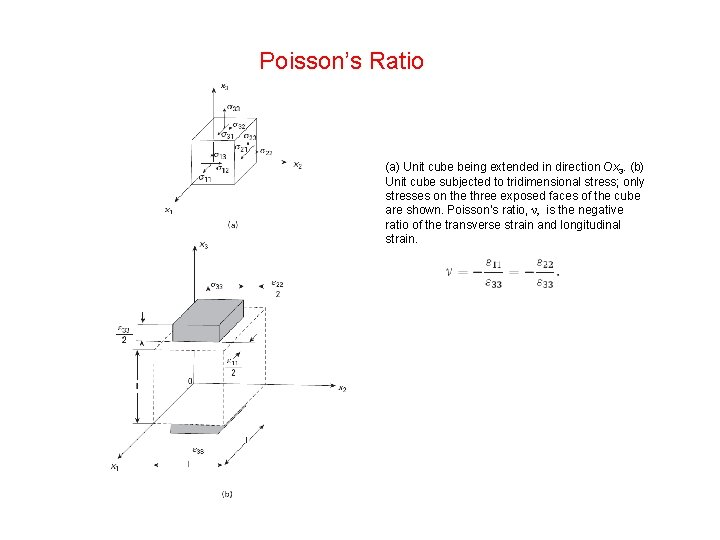Poisson’s Ratio (a) Unit cube being extended in direction Ox 3. (b) Unit cube subjected to tridimensional stress; only stresses on the three exposed faces of the cube are shown. Poisson’s ratio, ν, is the negative ratio of the transverse strain and longitudinal strain.Generalized Hooke's LawMohr Circle (a) Biaxial (or bidimensional) state of stress. (b) Mohr circle construction, general orientation (c) Mohr circle and construction, principal stresses and maximum shear stresses (Method I).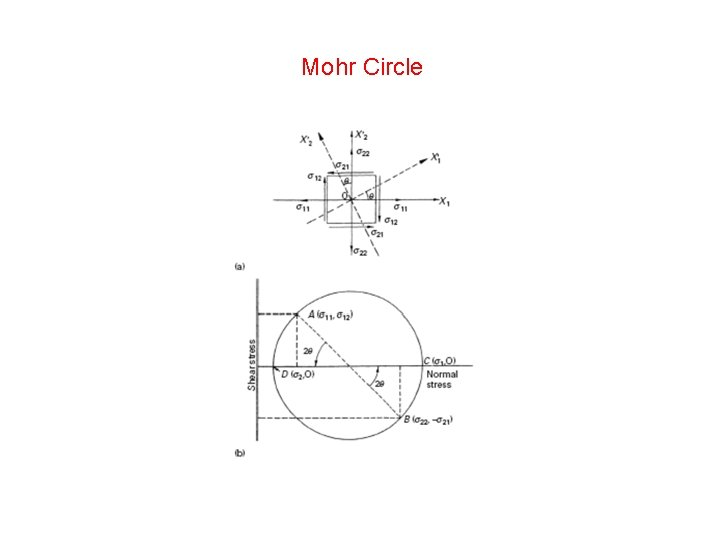Mohr CirclePure ShearHooke’s Law for Anisotropic Materials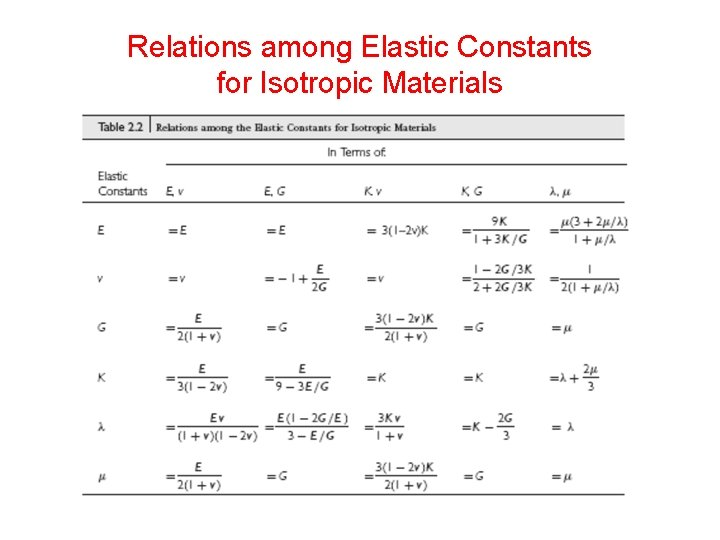Relations among Elastic Constants for Isotropic Materials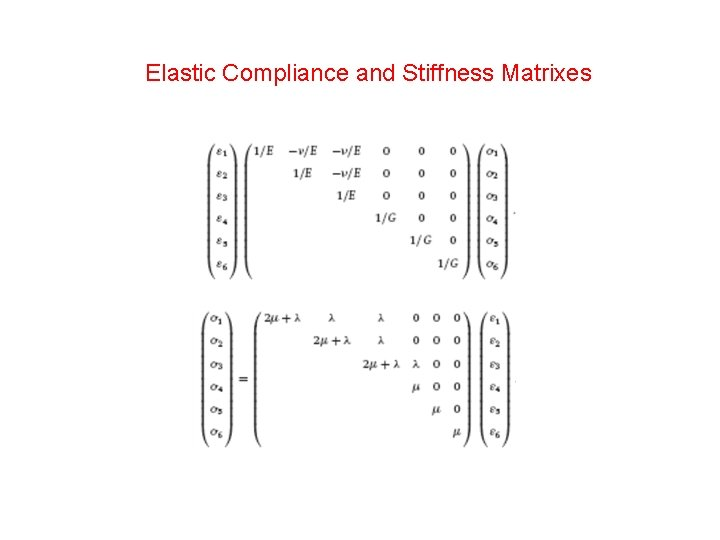Elastic Compliance and Stiffness MatrixesCompliance Matrix for a Cubic System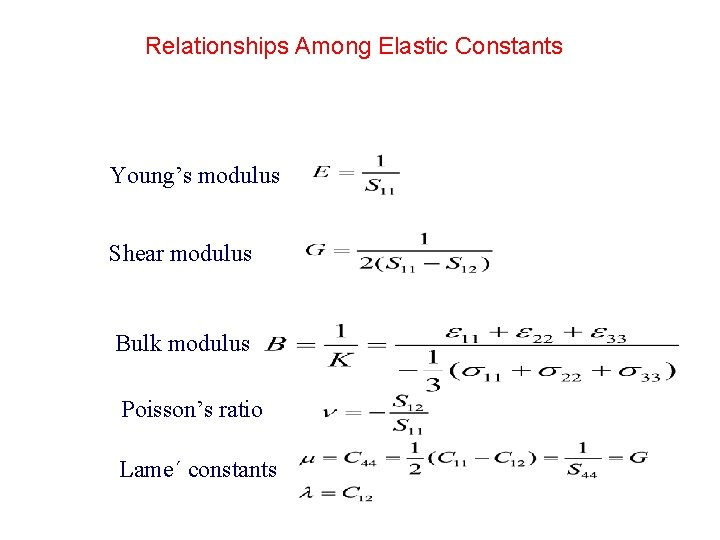Relationships Among Elastic Constants Young’s modulus Shear modulus Bulk modulus Poisson’s ratio Lame΄ constants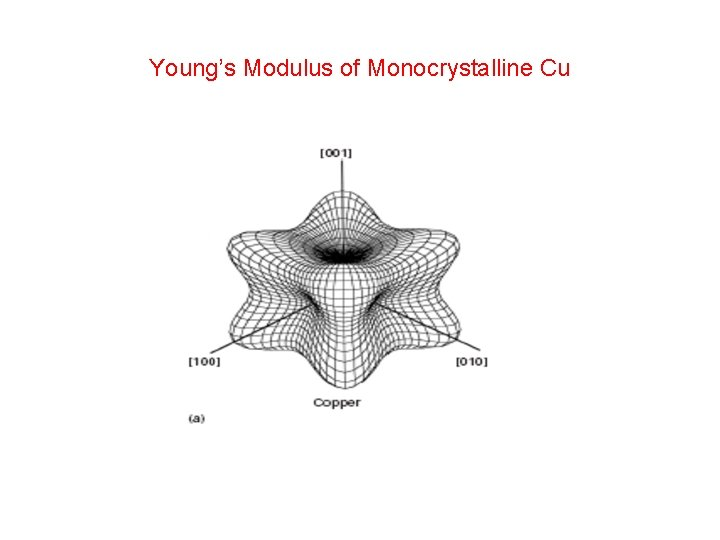Young’s Modulus of Monocrystalline Cu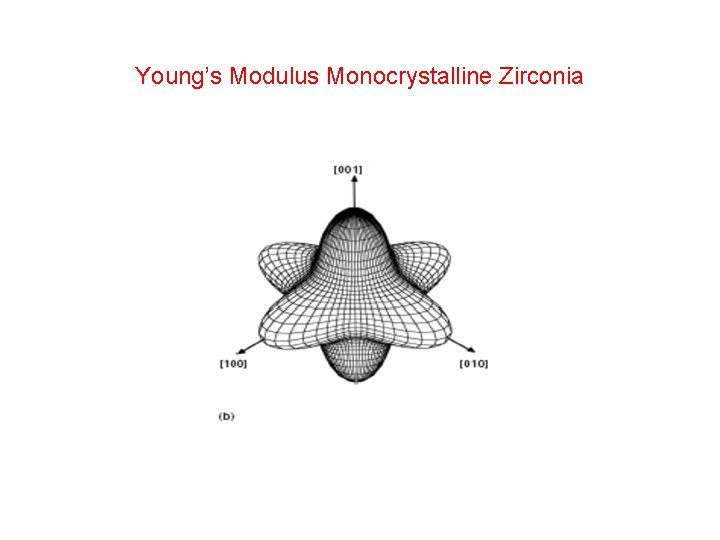Young’s Modulus Monocrystalline Zirconia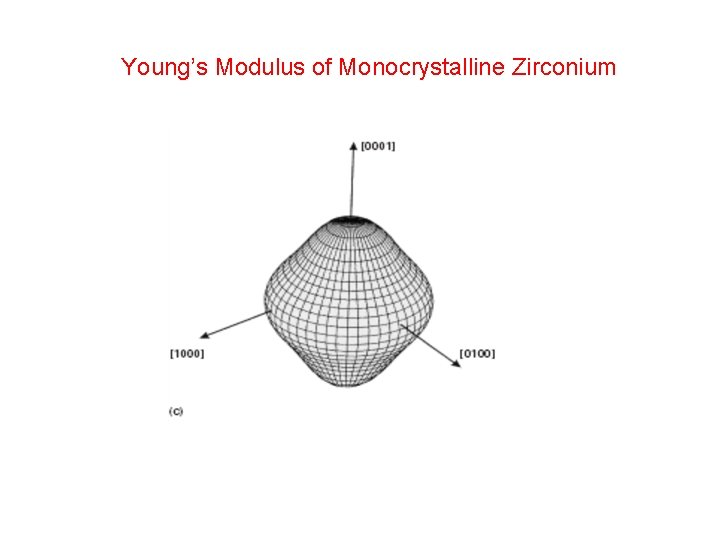Young’s Modulus of Monocrystalline Zirconium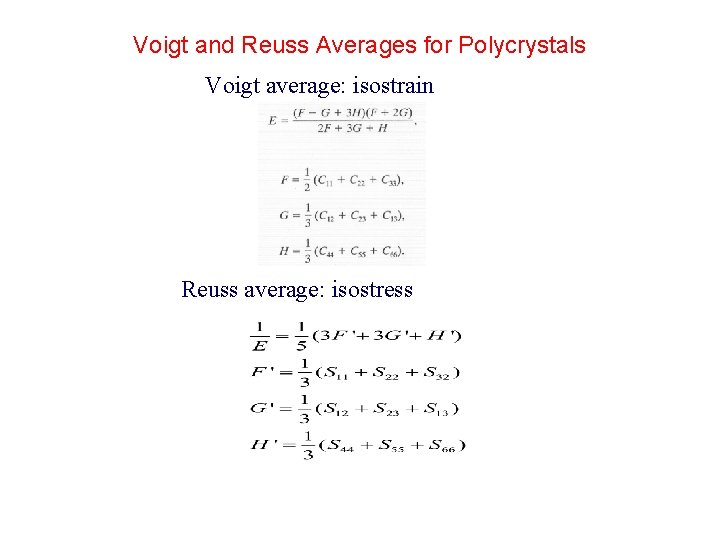Voigt and Reuss Averages for Polycrystals Voigt average: isostrain Reuss average: isostress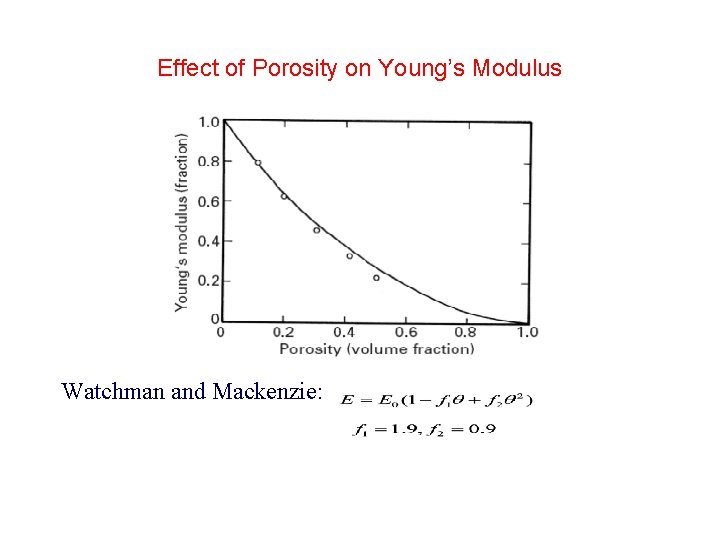Effect of Porosity on Young’s Modulus Watchman and Mackenzie:Effect of Microcracks on Young’s ModulusEffect of Microcracks on Young’s Modulus Salganik model O’connell & Budiansky modelYoung’s Modulus of PolymersYoung’s Modulus of Polymers as a Function of TemperatureViscoelasticity n = 0: plastic n = 1: linear viscous (Newtonian) n ≠ 1 : nonlinear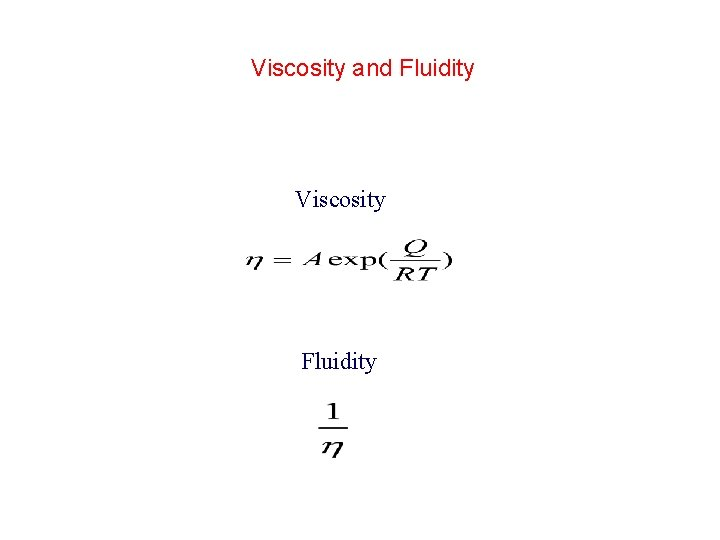Viscosity and Fluidity Viscosity Fluidity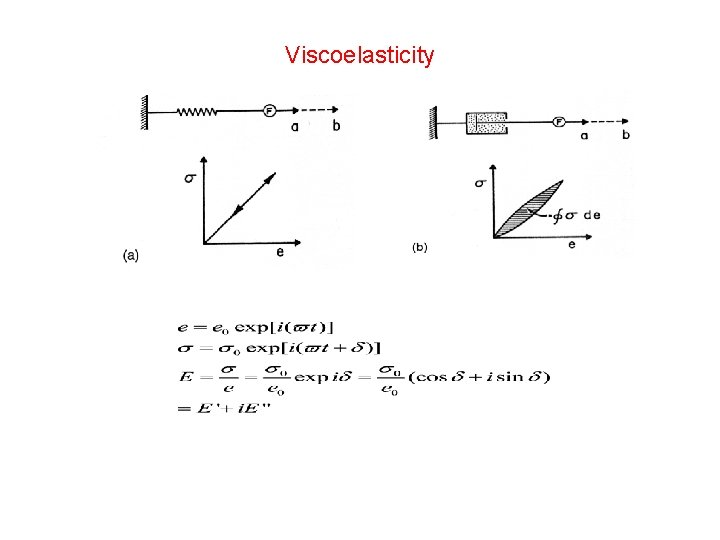Viscoelasticity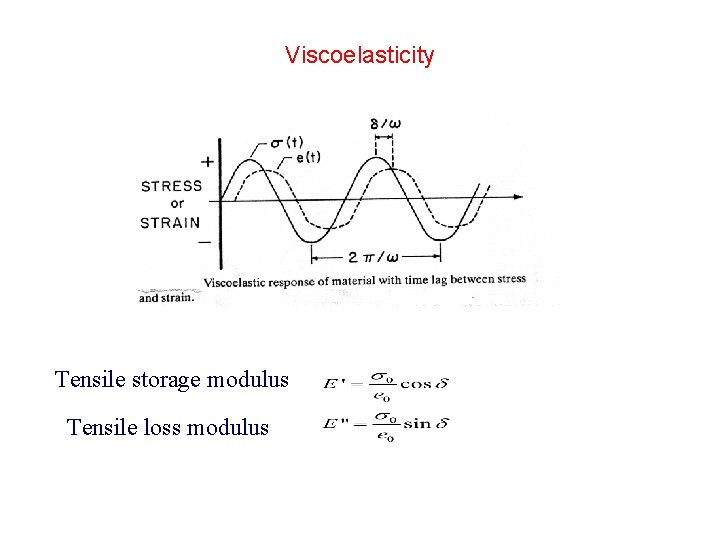Viscoelasticity Tensile storage modulus Tensile loss modulus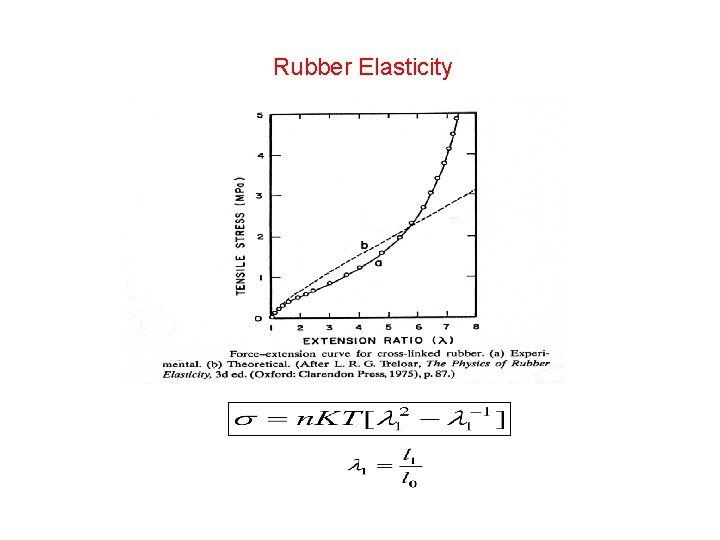Rubber ElasticityStress-Strain Behavior of Biological Materials (a) Stress–strain response of human vena cava: circles-loading; squares-unloading. (Adapted from Y. C. Fung, Biomechanics (New York: Springer, 1993), p. 366. ) (b) Representation of mechanical response in terms of tangent modulus (slope of stress–strain curve) vs. stress. (Adapted from Y. C. Fung. Biomechanics, New York: Springer, 1993), p. 329. )Residual Stresses in ArteriesCartilage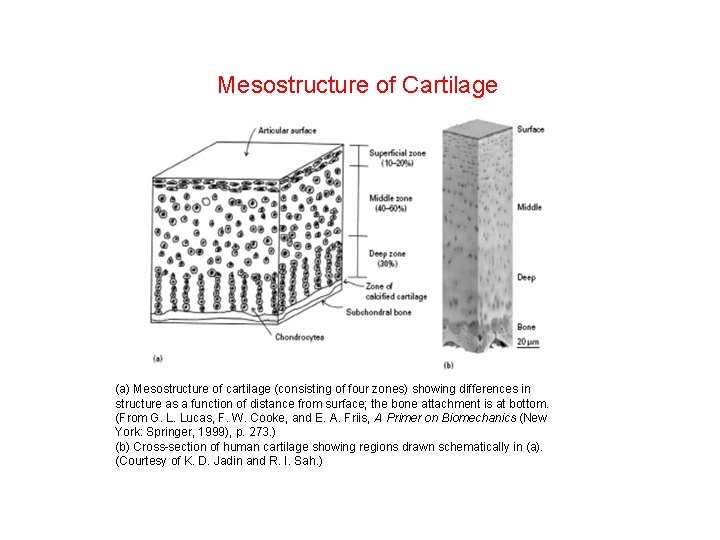Mesostructure of Cartilage (a) Mesostructure of cartilage (consisting of four zones) showing differences in structure as a function of distance from surface; the bone attachment is at bottom. (From G. L. Lucas, F. W. Cooke, and E. A. Friis, A Primer on Biomechanics (New York: Springer, 1999), p. 273. ) (b) Cross-section of human cartilage showing regions drawn schematically in (a). (Courtesy of K. D. Jadin and R. I. Sah. )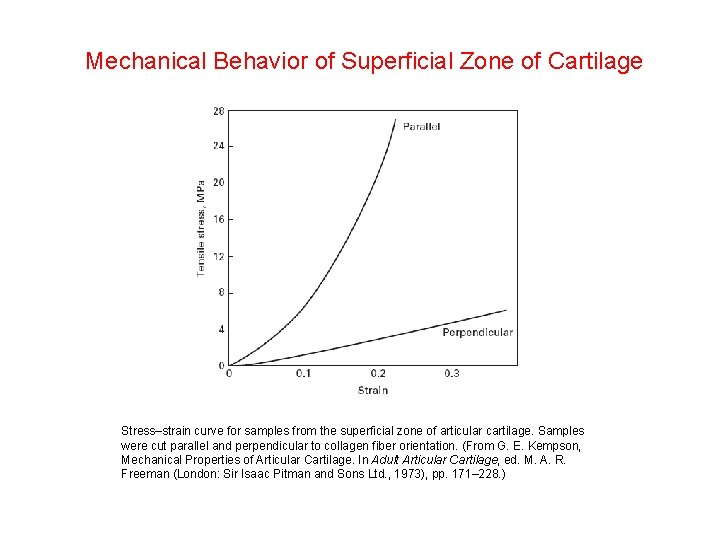Mechanical Behavior of Superficial Zone of Cartilage Stress–strain curve for samples from the superficial zone of articular cartilage. Samples were cut parallel and perpendicular to collagen fiber orientation. (From G. E. Kempson, Mechanical Properties of Articular Cartilage. In Adult Articular Cartilage, ed. M. A. R. Freeman (London: Sir Isaac Pitman and Sons Ltd. , 1973), pp. 171– 228. )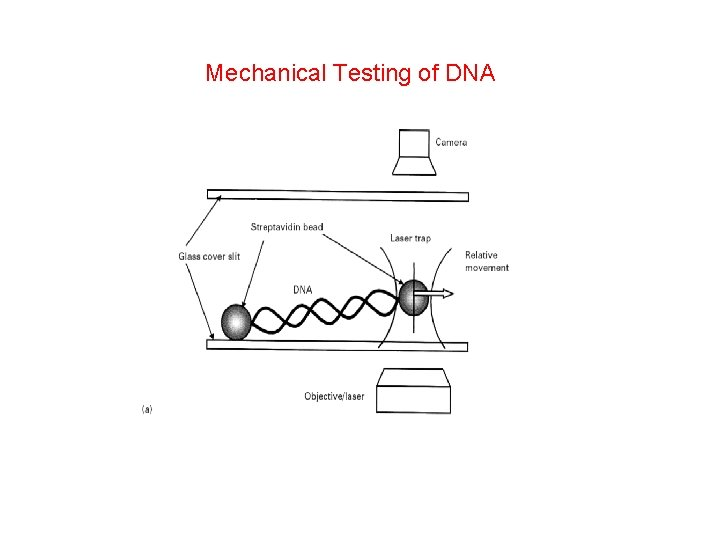Mechanical Testing of DNAForce vs. Extension for DNA MoleculeStresses in a Thin Film Effect of stresses in a thin film on bending of substrate; (a) tensile stresses in thin film; (b) compressive stresses in thin film.Elastic Constant and Bonding Two atoms with an imaginary spring between them; (a) equilibrium position; (b) stretched configuration under tensile force; (c) compressed configuration under compressive force.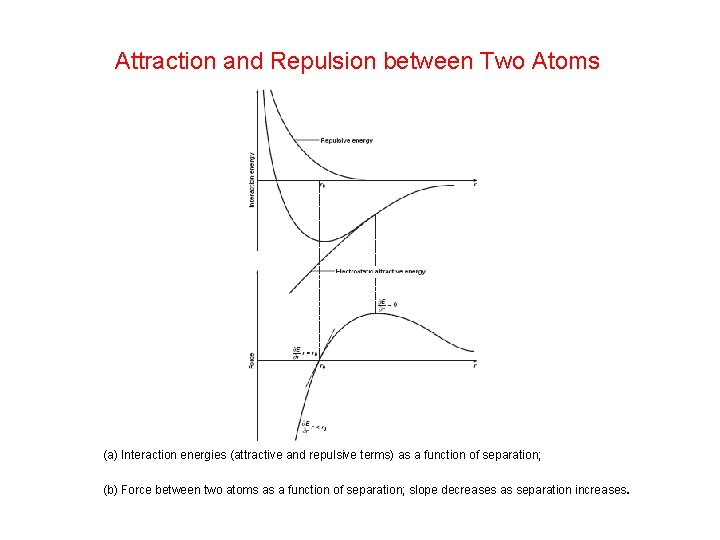Attraction and Repulsion between Two Atoms (a) Interaction energies (attractive and repulsive terms) as a function of separation; (b) Force between two atoms as a function of separation; slope decreases as separation increases.